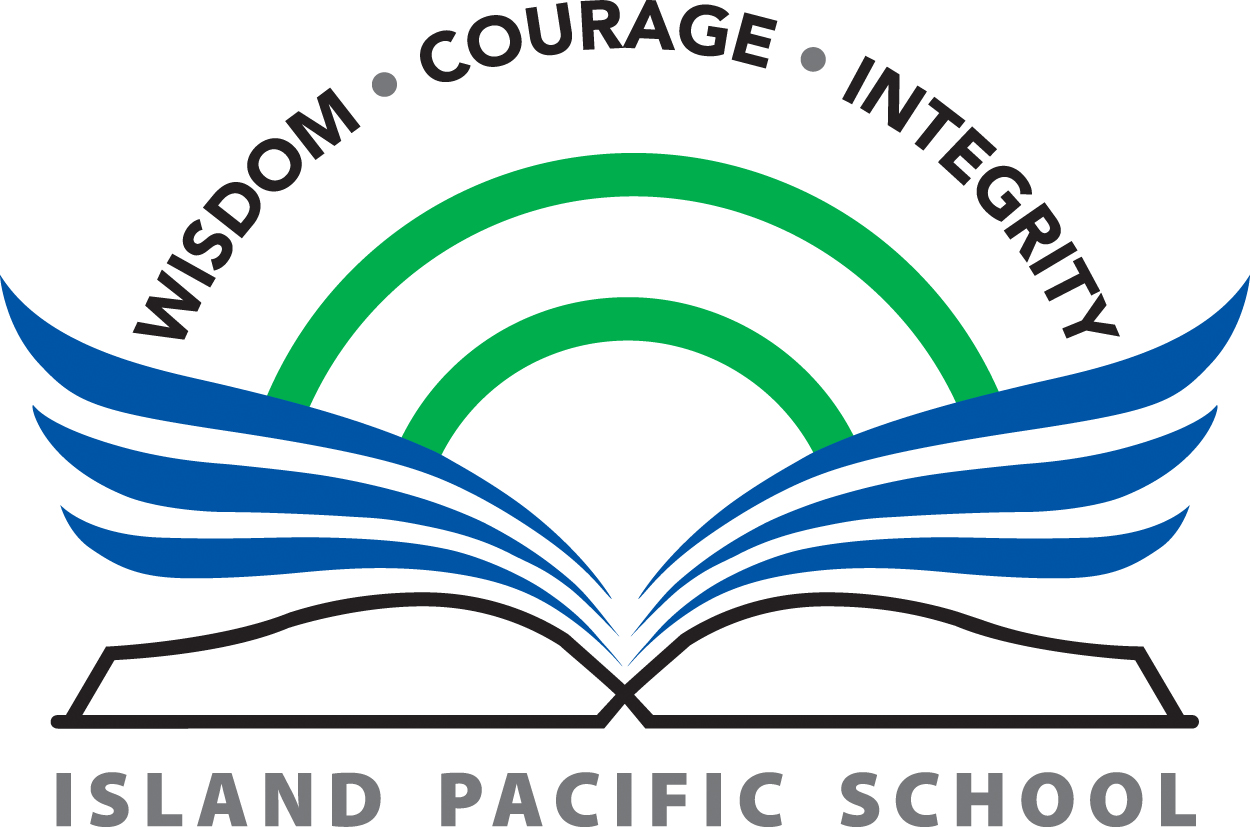COURSE OUTLINE  2019-2020

COURSE:       Mathematics 6
TEACHER:         Jennifer Henrichsen
CONTACT:        jhenrichsen@go.islandpacific.org

Course Description

There are three central goals within our middle school math program. These goals are complementary and dependent on each other. Although attention to these goals is interwoven throughout most programs, there seems to be a logical progression that leads from one to the other. A student must first and foremost be proficient in the skills of the different areas of the math program. It is only through practice, familiarity and success that a student then develops the attitude to confidently and willingly attempt mathematical problems. When both the skills and attitude have developed, the student is prepared to open the door and appreciate the role math can play to better understand the world.

There is an established set of skills that students need to be proficient in at each level in a math program. These skills can be grouped as Number Concepts, Patterns and Relations, Shape and Space, and Statistics and Probability.  A historical perspective is discussed at relative points to put the learning in context. It is my hope that these students will develop a strong skill set that will let them confidently approach the questions and investigations that the world of math has to offer.

The aims of MYP mathematics courses are to encourage and enable students to:

• enjoy mathematics, develop curiosity and begin to appreciate its elegance and power
• develop an understanding of the principles and nature of mathematics
• communicate clearly and confidently in a variety of contexts
• develop logical, critical and creative thinking
• develop confidence, perseverance and independence in mathematical thinking and problem-solving
• develop powers of generalization and abstraction
• apply and transfer skills to a wide range of real-life situations, other areas of knowledge and future developments
• appreciate how developments in technology and mathematics have influenced each other; the moral, social and ethical implications arising from the work of mathematicians and the applications of mathematics; the international dimension in mathematics; and the contribution of mathematics to other areas of knowledge
• develop the knowledge, skills and attitudes necessary to pursue further studies in mathematics
• develop the ability to reflect critically upon their own work and the work of others.

Year Overview  - these topics will be covered throughout the year

Number Concepts
decimal operations

percents 1-100%, percent of a number
decimal, fraction, and percent relationships
fractions - ordering, converting mixed to improper

order of operations

Patterns and Relations

tables of values to solve problems

graphs of linear relations

Equations
algebraic expressions and equations
one step linear equations

Shape and Space

understanding angles- identify and measure

sums of interior angles

perimeter and area

construct and compare triangles

transformations on the Cartesian Plane

Statistics and Probability
collecting data appropriately

data analysis- central tendency, outliers and range

create and interpret line graphs, bar graphs and circle graphs

ratios, fractions and percents to express probabilities

Homework

At Island Pacific School we encourage students to make good use of their Homeroom Study blocks and time allotted in class to complete various assignments. In this particular course, I encourage students to practice their math skills as often as possible. Extra work will be given based on individual needs, and the unit of study. They will need more time to complete projects and review for tests.

Resources & Materials

In this course we will use a combination of resources. We will use the text Math Makes Sense, elements of the JUMP Math program and on-line resources (www.IXL.com). Regular course work will be augmented by project based units of inquiry, problem solving from University of Waterloo Math Competitions, and web resources.

Students should keep their workbooks and other materials in a 3 hole binder. They will need pencils, pens, lined paper, erasers for this course. They should also have a math set with protractor, ruler and compass and a scientific calculator.

Assessment

Island Pacific School uses the International Baccalaureate rubrics to evaluate students in reference to six distinct criteria. In this course, those criteria are as follows:

Criterion A:  Knowledge and Understanding

Criterion B: Investigating Patterns

Criterion C: Communication in Mathematics

Criterion D: Mathematics in Real Life Context

 For a complete list of the Mathematics criteria and their relevant level descriptors for Year 1 and 2, you can download a PDF at the following URLs: Year 1 and 2: http://goo.gl/4aMl35For a complete description of BC’s New Curriculum Core Competencies for https://curriculum.gov.bc.ca/curriculum/mathematics/6